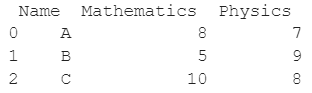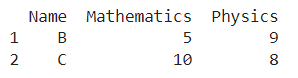# How to delete DataFrame Row In Pandas Based On Column Value?

A DataFrame is the primary data structure of the Pandas library and is commonly used for storing and working with tabular data. A common operation that could be performed on such data is deleting a DataFrame row in Pandas based on column value to remove unwanted information from the dataframe.

To start working with Pandas, we first need to import this statement in the Python code :

### Python 3 Code :

``import pandas as pd``

## Running Example

Let us understand this operation with the help of an example. Consider the following DataFrame containing 3 students with names A, B and C and their corresponding marks (out of 10) for two subjects, Mathematics and Physics.Python code snippet for generating the above DataFrame :

### Python 3 Code :

``````# Importing pandas
import pandas as pd

# Dictionary for our data
data = {'Name' : ['A', 'B', 'C'], 'Mathematics' : [8, 5, 10], 'Physics' : [7, 9, 8]}

# DataFrame for the dictionary
df = pd.DataFrame(data)

# Printing the DataFrame
print(df)``````

Here, data is a dictionary we created to initialize the DataFrame. For this, we use the DataFrame() function of the Pandas library which takes the dictionary as an argument and returns the required DataFrame.

Now, let’s say we need to remove the rows of students that have received less than 8 marks in the subject physics. Thus, the student in the first row will be deleted and the resulting DataFrame would look like this :Let us look at different ways of performing this operation on a given DataFrame :

## Remove DataFrame Rows by Selecting required Rows Only

This method is pretty straightforward and is the most commonly used one. In this method, we select the required rows instead of deleting the ones that are not required.

So, instead of deleting the rows with less than 8 marks in physics, we select the rows with greater than or equal to 8 marks in physics.

This operation is often referred to as boolean masking. Here, df[‘Physics’] is used to access the column with the label Physics in the dataframe. The changes are not made in place so we need to reassign the column.

Let us look at the Python code and corresponding output for this method.

### Python 3 Code :

``````import pandas as pd

# Dictionary for our data
data = {'Name' : ['A', 'B', 'C'], 'Mathematics' : [8, 5, 10], 'Physics' : [7, 9, 8]}

# DataFrame for the dictionary
df = pd.DataFrame(data)

# Selecting the required rows
df = df[df['Physics'] >= 8]

# Printing the updated DataFrame
print(df)``````

Output :Another way to perform the same operation would be to use df.Physics to access the column labeled as Physics instead of using df[‘Physics’]. The changes are not made in place so we need to reassign the column. Let us look at the Python code and corresponding output for this method -

### Python 3 Code :

``````#Â ImportingÂ pandas
importÂ pandasÂ asÂ pd

# Dictionary for our data
data = {'Name' : ['A', 'B', 'C'], 'Mathematics' : [8, 5, 10], 'Physics' : [7, 9, 8]}

# DataFrame for the dictionary
df = pd.DataFrame(data)

# Selecting the required rows
df = df[df.Physics >= 8]

# Printing the updated DataFrame
print(df)``````

Output :## Remove DataFrame Rows by Using the dataframe.drop() function

In this method, we use the dataframe.drop() function with the index of rows to be dropped, the first one here, passed as a parameter.

Here, df[‘Physics’] is used to access the column with the label Physics in the dataframe. After selecting the rows to be deleted, i.e the ones with less than 8 marks in physics, we want to get the index of these rows.

For this, we use the dataframe.index property, which returns 0 in this case, the index of the first row. The changes are not made in place so we need to reassign the column.

Let us look at the python code and corresponding output for this method.

### Python 3 Code :

``````#Â ImportingÂ pandas
importÂ pandasÂ asÂ pd

# Dictionary for our data
data = {'Name' : ['A', 'B', 'C'], 'Mathematics' : [8, 5, 10], 'Physics' : [7, 9, 8]}

# DataFrame for the dictionary
df = pd.DataFrame(data)

# Selecting the required rows
df = df.drop(df[df['Physics'] < 8].index)

# Printing the updated DataFrame
print(df)``````

Output :Another way to perform the same operation would be to use df.Physics to access the column labeled as Physics instead of using df[‘Physics’]. Let us look at the Python code and corresponding output for this method -

### Python 3 Code :

``````#Â ImportingÂ pandas
importÂ pandasÂ asÂ pd

# Dictionary for our data
data = {'Name' : ['A', 'B', 'C'], 'Mathematics' : [8, 5, 10], 'Physics' : [7, 9, 8]}

# DataFrame for the dictionary
df = pd.DataFrame(data)

# Selecting the required rows
df = df.drop(df[df.Physics < 8].index)

# Printing the updated DataFrame
print(df)``````

Output :If we want to make the changes in place to avoid reassigning the dataframe, we need to pass the parameter inplace as True. Let us look at the python code and corresponding output for this method.

### Python 3 Code :

``````#Â ImportingÂ pandas
importÂ pandasÂ asÂ pd

# Dictionary for our data
data = {'Name' : ['A', 'B', 'C'], 'Mathematics' : [8, 5, 10], 'Physics' : [7, 9, 8]}

# DataFrame for the dictionary
df = pd.DataFrame(data)

# Selecting the required rows
df.drop(df[df['Physics'] < 8].index, inplace = True)

# Printing the updated DataFrame
print(df)``````

Output :Another way to perform the same operation would be to use df.Physics to access the column labeled as Physics instead of using df[‘Physics’] along with using inplace = True parameter. Let us look at the Python code and corresponding output for this method -

### Python 3 Code :

``````#Â ImportingÂ pandas
importÂ pandasÂ asÂ pd

# Dictionary for our data
data = {'Name' : ['A', 'B', 'C'], 'Mathematics' : [8, 5, 10], 'Physics' : [7, 9, 8]}

# DataFrame for the dictionary
df = pd.DataFrame(data)

# Selecting the required rows
df.drop(df[df.Physics < 8].index, inplace = True)

# Printing the updated DataFrame
print(df)``````

Output :## Conclusion

In this topic, we have learned how to delete a DataFrame row in Pandas based on column value from an existing Pandas DataFrame, following a running example of test scores of students in different subjects, thus giving us an intuition of how this concept could be applied in real-world situations. Feel free to reach out to info.javaexercise@gmail.com in case of any suggestions

Trending